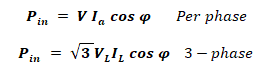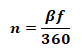# Synchronous, Stepper and AC Motors Formulas and Equations

## AC Machine Formulas & Equations for Stepper and Synchronous Motors

Following are the useful equations and AC motors formulas while designing and analyzing synchronous motors, stepper motors and other related AC machines.### Synchronous Machine:

#### Speed of Synchronous Machine:

Synchronous machine are designed to be operated at synchronous speed, which is given by:Where

• is the synchronous speed
• f is the line voltage frequency
• P is the number of poles in a machine

### Synchronous Motor:

#### Voltage Equation of Synchronous Motor:

V = Eb + Ia(Ra + jXs)

Where

#### Resultant Voltage:

The difference between the voltage applied V and back EMF is known as resultant voltage ER

ER = V – Eb

ER = Ia(Ra + jXs)

#### Internal Angle:

It is the angle by which the armature current Ia lags behind the resultant voltage in armature ER, and it is given by;#### Back EMF Generated:

E= KaφaNs

Where

• K= constant of the armature winding
• φ= magnetic Flux per pole of the rotor
• N= synchronous speed of the rotor

Related Posts:

#### Different Excitations:

• E= V             Normal Excitation                            Lagging Power Factor
• Eb < V             Under-Excitation                              Lagging Power Factor
• Eb > V             Over- Excitation                                Leading Power Factor

#### Input Power:

The input power of synchronous motor is given by:Where

Φ is the angle between V and Ia

#### Mechanical Power In Rotor:

• α is the load angle between E­& V
• Φ is the angle between V & Ia
• Tis gross torque produced
• Nis the synchronous speed

Related Posts:

### Stepper Motors Formulas

#### Step Angle:

• β = step angle, the angle of rotation of the shaft with each pulse.
• Ns = number of stator poles or teeth
• Nr = number of rotor poles or teeth

#### Resolution Of Stepper Motor:

The number of steps required to complete one revolution, its given by;The higher the resolution, the higher the accuracy of the stepper motor.

#### Motor Speed:Where

• n = motor speed in revolution per second
• f = stepping pulse frequency

Related Formulas and Equations Posts: